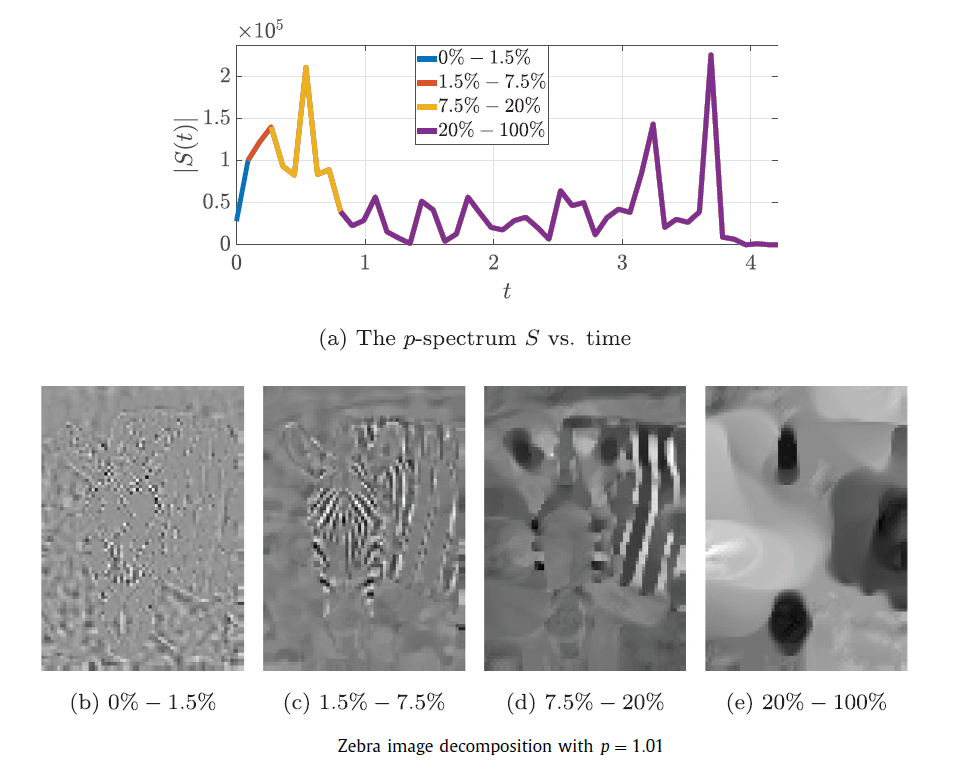# Ido Cohen and Guy Gilboa Signal Processing

In this work we develop a nonlinear decomposition, associated with nonlinear eigenfunctions of the p-Laplacian for p ∈ (1, 2). With this decomposition we can process signals of different degrees of smoothness.

We first analyze solutions of scale spaces, generated by γ-homogeneous operators, γ∈R. An analytic solution is formulated when the scale space is initialized with a nonlinear eigenfunction of the respective operator. We show that the flow is extinct in finite time for γ ∈ [0, 1).

A main innovation in this study is concerned with operators of fractional homogeneity, which require the mathematical framework of fractional calculus. The proposed transform rigorously defines the notions of decomposition, reconstruction, filtering and spectrum. The theory is applied to the p-Laplacian operator, where the tools developed in this framework are demonstrated.